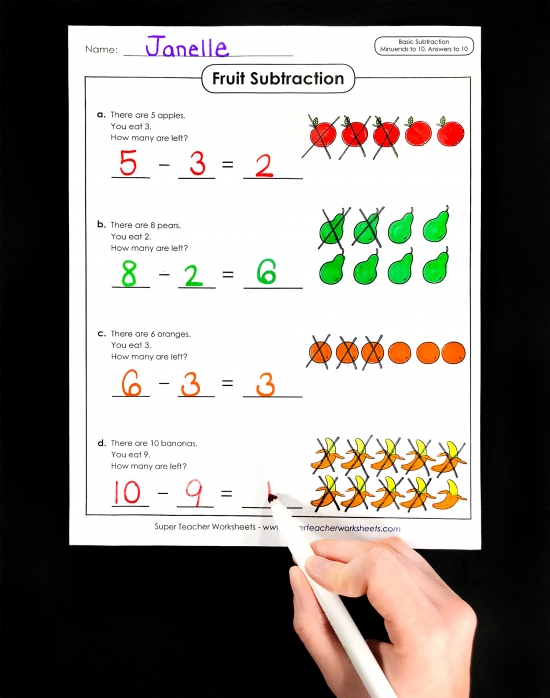# Basic Subtraction Activities for ChildrenBrowse our complete collection of basic subtraction worksheets for kids! You'll find resources such as subtraction matching, subtraction bingo, subtraction dice, subtraction word problems, subtraction star puzzles, subtraction cut-and-glue activities, number line subtraction, subtraction mystery pictures, a basic subtraction worksheets generator, speed drill worksheets, and subtraction fact practice. Visit our collection today!